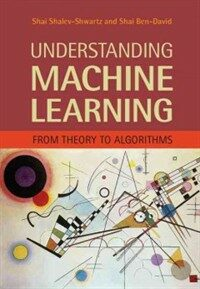> Detail View

# Detail View## Understanding machine learning : from theory to algorithms (Loan 1 times)

Material type
단행본
Personal Author
Shalev-Shwartz, Shai. Ben-David, Shai.
Title Statement
Understanding machine learning : from theory to algorithms / Shai Shalev-Shwartz, The Hebrew University, Jerusalem, Shai Ben-David, University of Waterloo, Canada.
Publication, Distribution, etc
New York, NY, USA :   Cambridge University Press,   c2014.
Physical Medium
xvi, 397 p. : ill. ; 26 cm.
ISBN
9781107057135 (hardback) 1107057132 (hardback)
요약
"Machine learning is one of the fastest growing areas of computer science, with far-reaching applications. The aim of this textbook is to introduce machine learning, and the algorithmic paradigms it offers, in a principled way. The book provides an extensive theoretical account of the fundamental ideas underlying machine learning and the mathematical derivations that transform these principles into practical algorithms. Following a presentation of the basics of the field, the book covers a wide array of central topics that have not been addressed by previous textbooks. These include a discussion of the computational complexity of learning and the concepts of convexity and stability; important algorithmic paradigms including stochastic gradient descent, neural networks, and structured output learning; and emerging theoretical concepts such as the PAC-Bayes approach and compression-based bounds. Designed for an advanced undergraduate or beginning graduate course, the text makes the fundamentals and algorithms of machine learning accessible to students and non-expert readers in statistics, computer science, mathematics, and engineering"--
Content Notes
Machine generated contents note: 1. Introduction; Part I. Foundations: 2. A gentle start; 3. A formal learning model; 4. Learning via uniform convergence; 5. The bias-complexity tradeoff; 6. The VC-dimension; 7. Non-uniform learnability; 8. The runtime of learning; Part II. From Theory to Algorithms: 9. Linear predictors; 10. Boosting; 11. Model selection and validation; 12. Convex learning problems; 13. Regularization and stability; 14. Stochastic gradient descent; 15. Support vector machines; 16. Kernel methods; 17. Multiclass, ranking, and complex prediction problems; 18. Decision trees; 19. Nearest neighbor; 20. Neural networks; Part III. Additional Learning Models: 21. Online learning; 22. Clustering; 23. Dimensionality reduction; 24. Generative models; 25. Feature selection and generation; Part IV. Advanced Theory: 26. Rademacher complexities; 27. Covering numbers; 28. Proof of the fundamental theorem of learning theory; 29. Multiclass learnability; 30. Compression bounds; 31. PAC-Bayes; Appendix A. Technical lemmas; Appendix B. Measure concentration; Appendix C. Linear algebra.
Bibliography, Etc. Note
Includes bibliographical references (p. 385-393) and index.
Machine learning. Algorithms.

### Holdings Information

No. Location Call Number Accession No. Availability Due Date Make a Reservation Service
No. 1 Location Call Number 006.31 S528u Accession No. 121246195 Availability Available Due Date Make a Reservation Service

### Contents information

`Introduction -- I. Foundations -- A gentle start -- A formal learning model -- Learning via uniform convergence -- The bias-complexity tradeoff -- The VC-dimension -- Nonuniform learnability -- The runtime of learning -- II. From Theory to Algorithms -- Linear predictors -- Boosting -- Model selection and validation -- Convex learning problems -- Regularization and stability -- Stochastic gradient descent -- Support vector machines -- Kernel methods -- Multiclass, ranking, and complex prediction problems -- Decision trees -- Nearest neighbor -- Neural networks -- III. Additional Learning Models -- Online learning -- Clustering -- Dimensionality reduction -- Generative models -- Feature selection and generation -- IV. Advanced Theory -- Rademacher complexities -- Covering numbers -- Proof of the fundamental theorem of learning theory -- Multiclass learnability -- Compression bounds -- PAC-Bayes.`

### New Arrivals Books in Related Fields

최성환 (2020)

#### (주머니 속의) 머신러닝

Harrison, Matt (2021)

조준모 (2021)

한선관 (2021)

#### 데이터 분석과 인공지능 활용

데이터분석과인공지능활용편찬위원회 (2021)

고경희 (2021)

김선영 (2021)

주해종 (2021)

임화연 (2020)

김대용 (2021)

심준식 (2021)

차병래 (2021)

김운아 (2021)

이영호 (2021)

#### 3시간에 배우는 인공지능 데이터 분석, 오렌지 : 문과 출신 비개발자 비즈니스맨을 위한

서울과학종합대학원. 디지털혁신처 (2021)

멀티코어 (2021)

#### Efficient processing of deep neural networks

Sze, Vivienne (2020)

#### Deep learning with PyTorch

Stevens, Eli (2020)

#### Fundamentals of machine learning for predictive data analytics : algorithms, worked examples, and case studies / 2nd ed

Kelleher, John D. (2020)